Next: Regularizing effect revisited () Up: Kinetic Formulations and Regularity Previous: Regularizing effect in one-space

# Velocity averaging lemmas (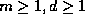)

We deal with solutions to transport equations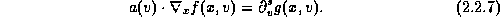The averaging lemmas, , , , state that in the generic non-degenerate case, averaging over the velocity space,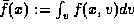, yields a gain of spatial regularity. The prototype statement reads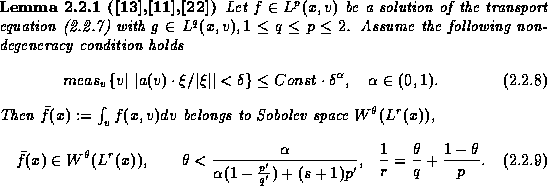Variants of the averaging lemmas were used by DiPerna and Lions to construct global weak (renormalized) solutions of Boltzmann, Vlasov-Maxwell and related kinetic systems, , ; in Bardos et. al., , averaging lemmas were used to construct solutions of the incompressible Navier-Stokes equations. We turn our attention to their use in the context of nonlinear conservation laws and related equations.

Proof.(Sketch). We shall sketch the proof in the particular case, p=q which will suffice to demonstrate the general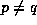case.

Let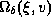denote the set where the symbol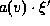is 'small',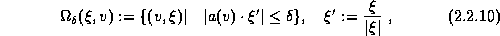and decompose the average,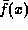accordingly: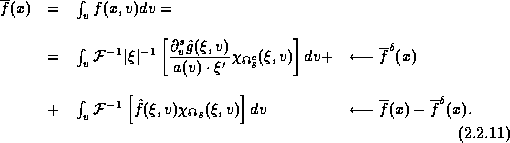Here,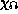represents the usual smooth partitioning relative to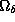and its complement,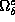. On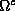, the symbol is 'bounded away' from zero, so we gain one derivative: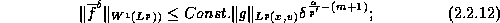On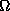- along the `non uniformly elliptic' rays, we have no gain of regularity, but instead, our non-degeneracy assumption implies that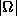is a 'small' set and therefore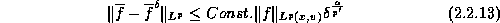Both (2.2.12) and (2.2.13) are straightforward for p=2 and by estimating the corresponding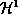multipliers, the case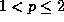follows by interpolation. Finally, we consider the K-functional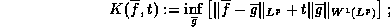The behavior of this functional,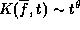, characterize the smoothness of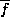in the intermediate space between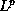and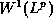: more precisely,belongs to Besov space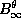with 'intermediate' smoothness of order.
Now set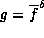, then with appropriately scaledwe find thatwith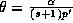. This means that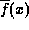belongs to Besov space,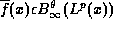and (2.2.9) (with p=q=r) follows.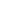Remark. In the limiting case of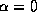in (2.2.8), one finds that if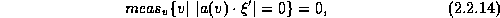then averaging is a compact mapping,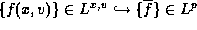. The case p=2 follows from Gèrard's results .Next: Regularizing effect revisited () Up: Kinetic Formulations and Regularity Previous: Regularizing effect in one-space